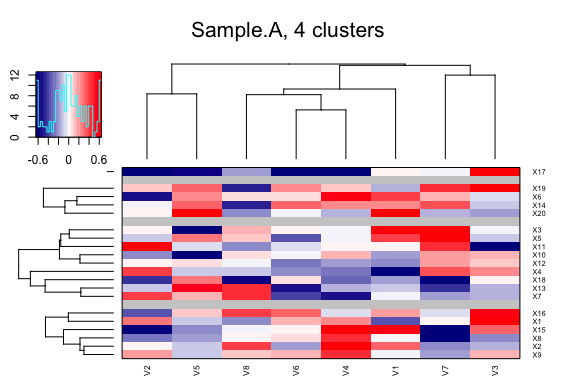Generate Heatmaps Based on Partitioning Around Medoids (PAM)

library(PAMhm)
#>
#> Attaching package: 'BiocGenerics'
#> The following objects are masked from 'package:parallel':
#>
#>     clusterApply, clusterApplyLB, clusterCall, clusterEvalQ,
#>     clusterExport, clusterMap, parApply, parCapply, parLapply,
#>     parLapplyLB, parRapply, parSapply, parSapplyLB
#> The following objects are masked from 'package:stats':
#>
#>     IQR, mad, sd, var, xtabs
#> The following objects are masked from 'package:base':
#>
#>     Filter, Find, Map, Position, Reduce, anyDuplicated, append,
#>     as.data.frame, basename, cbind, colnames, dirname, do.call,
#>     duplicated, eval, evalq, get, grep, grepl, intersect, is.unsorted,
#>     lapply, mapply, match, mget, order, paste, pmax, pmax.int, pmin,
#>     pmin.int, rank, rbind, rownames, sapply, setdiff, sort, table,
#>     tapply, union, unique, unsplit, which, which.max, which.min
#> Welcome to Bioconductor
#>
#>     Vignettes contain introductory material; view with
#>     'browseVignettes()'. To cite Bioconductor, see
#>     'citation("Biobase")', and for packages 'citation("pkgname")'.

GPL-3

Description

Data are partitioned (clustered) into k clusters “around medoids”, which is a more robust version of K-means implemented in the function pam() in the ‘cluster’ package. The PAM algorithm is described in (Kaufman and Rousseeuw, 1990). Please refer to the pam() function documentation for more references. Clustered data is plotted as a split heatmap allowing visualisation of representative “group-clusters” (medoids) in the data as separated fractions of the graph while those “sub-clusters” are visualised as a traditional heatmap based on hierarchical clustering.

Installation

CRAN

install.packages("PAMhm")

Latest development version

install.packages("devtools")
devtools::install_github("vfey/PAMhm")

Usage

A simple example

Generate a random 10x10 matrix and plot it using default values. We use set.seed() for reproducibility.

set.seed(1234)
mat <- matrix(c(rnorm(100, mean = 2), rnorm(100, mean = -1.8)), nrow = 20)
PAM.hm(mat, cluster.number = 3)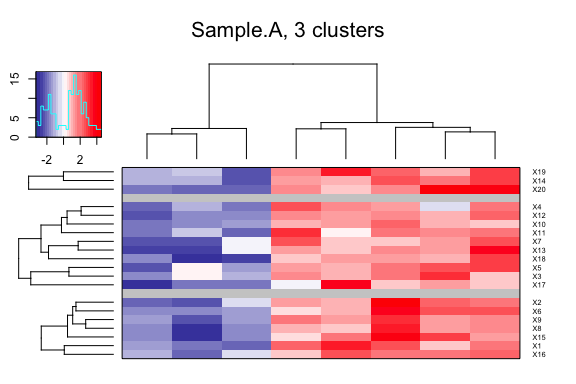A plot with more than one cluster number

The cluster number is given as a vector of numbers (numeric or character) used for PAM clustering (corresponds to argument k in cluster::pam). If it is provided as a character vector, this is broken down to a numeric vector accepting comma-separated strings in the form of, e.g, “4” and “2-5”. The clustering algorithm then iterates through all given numbers.

PAM.hm(mat, cluster.number = c("2", "4-5"))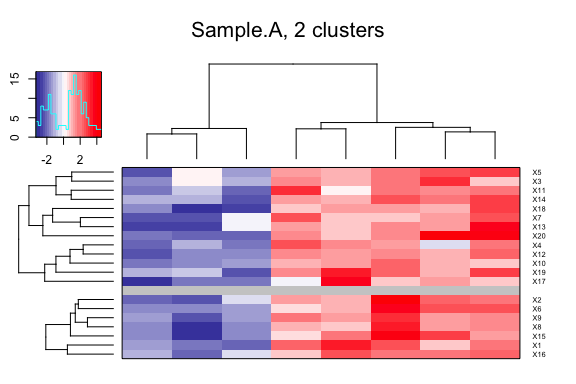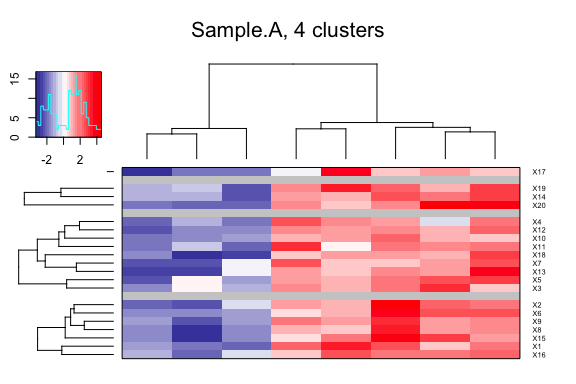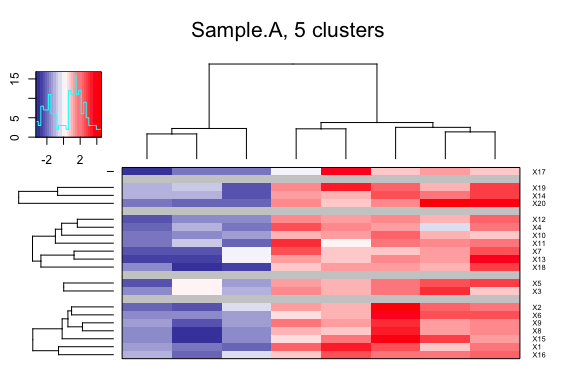A trimmed plot

If the data contains outliers, the heatmap may not be very informative as the extreme values distort the colour distribution.
One way to make this work is to “clean” the data by replacing extreme values at both ends of the distribution by less extreme values or, in other words, “shrinking outlying observations to the border of the main part of the data” (robustHD::winsorize), a process called winsorization and implemented in R packages robustHD used here, or DescTools, for example.
Instead of modifying your data matrix for a plot, PAM.hm accepts a trim value to “cut off” the data distribution internally before plotting. Values and both ends of the distribution, larger or smaller, respectively, will be made equal to +/-trim. There are two presets: trim = -1, which is the default (or any value smaller than 0) means that the smaller of the two largest absolute values at both ends of the winzorised distribution rounded to three digits will be used. trim = NULL means no trimming.
Note that trimming only makes sense for data containing both positive and negative values as the goal is to make the distribution symmetrical around zero to avoid over-emphasizing one side of the colour scheme. For that reason the matrix is internally winsorized by default and NOT trimmed and for data containing only positive or only negative values trimming is disabled.
To demonstrate the default trimming process, we calculate the trim value by using robustHD::winsorize on our matrix.

Plot without trimming

We introduce an outlier to the matrix to visualise the effect on the colour distribution.

mat <- mat * 20
PAM.hm(mat, cluster.number = 3, trim = NULL, winsorize.mat = FALSE)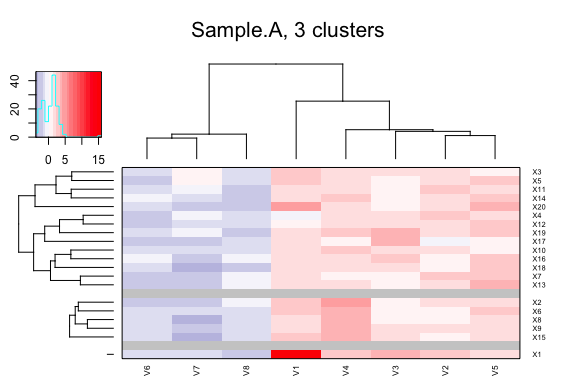The outlier clearly dominates the heatmap and all other values are pretty weak and almost indistinguishable. Let’s try with default trimming.

Plot with default (winzorized) trimming

We manually calculate the trim value by using winsorization on the matrix including the outlier and then apply the same algorithm used internally to obtain a value from a point slightly “shifted inside” the distribution.

m <- robustHD::winsorize(as.numeric(mat))
tr <- min(abs(round(c(min(m, na.rm = TRUE), max(m, na.rm = TRUE)), 3)), na.rm = TRUE)
PAM.hm(mat, cluster.number = 3, trim = tr)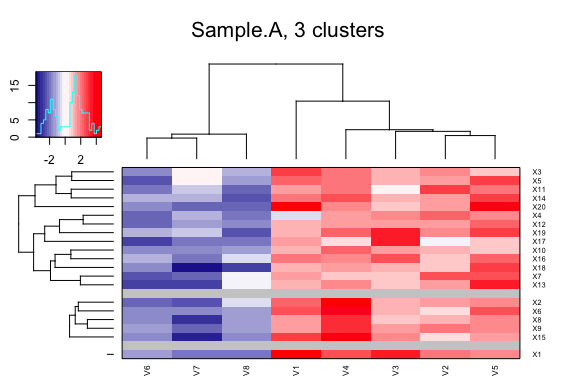We can see that the default trimming does a decent job on improving the readability of the heatmap. Note that the outlier is still clearly visible but was shrunk to the main distribution as can be seen in the colour key labels.

For some data it may be desirable to emphasize the lower range of the data. To achieve that winsorization can be disabled and trimming set to -1 which in that case defaults to the largest possible absolute integer, i.e., the smaller of the to extreme integers. Values at the borders of the distribution will be coloured identically but the colours depicting the middle range around 0 will be more fine-grained.

PAM.hm(mat, cluster.number = 3, trim = -1, winsorize.mat = FALSE)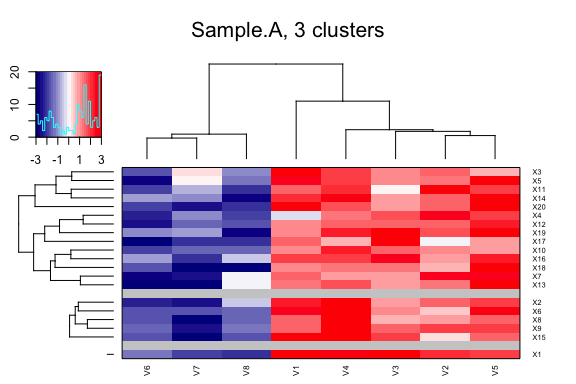Median-centering

To better visualise differences between the two extremes of a data distribution with only positive or negative values, data can be centered around the median. Median-centering is robust to outliers (as compared to mean-centering) and will ensure that the plotted distribution is centered around 0, so values smaller than the median of the original data are depicted in blue and values larger than the median are depicted in red (in the present colour scheme).
Centering can be done row-wise, column-wise or using the grand median across the entire matrix.

Row-wise centering without trimming

First, the positive-value matrix is plotted without centering or trimming.

set.seed(1234)
mat <- matrix(rnorm(200, mean = 3.6), nrow=20)
PAM.hm(mat)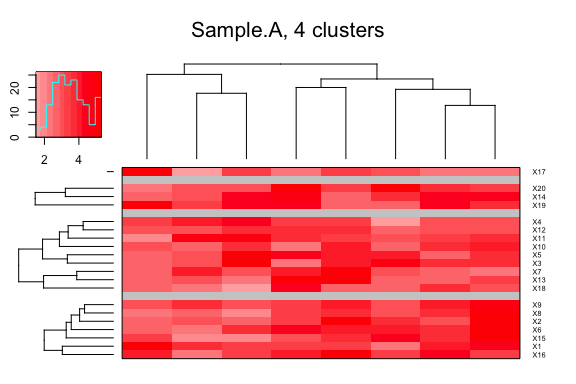This looks OK but we want to emphasize the smaller values in the matrix so we median-center each column and plot again. Note that we have to actively disable trimming and winsorization now to see the centering effect which is not a problem since data “cleaning” by trimming or winsorizing is not absolutely necessary because median-centering effectively arranges the data nearly symmetrical around 0.

PAM.hm(mat, medianCenter = "column", trim = NULL, winsorize.mat = FALSE)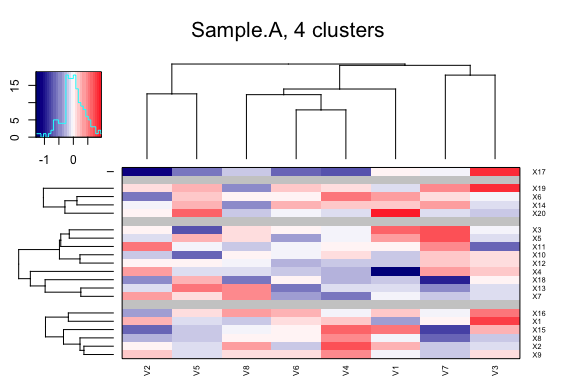If we now want to emphasize the finer differences in the range around 0 we enable automatic trimming.

PAM.hm(mat, medianCenter = "column", trim = -1)